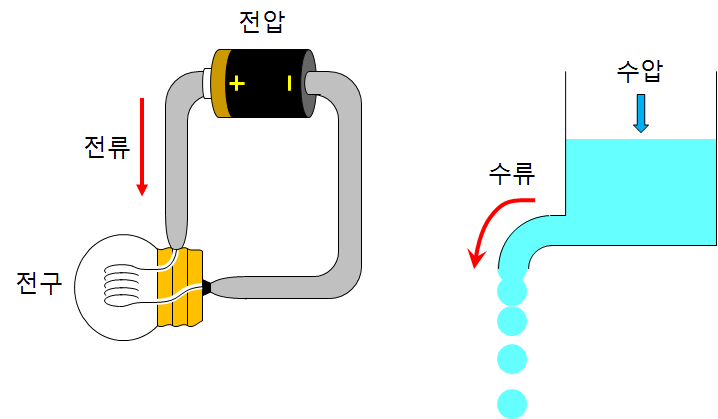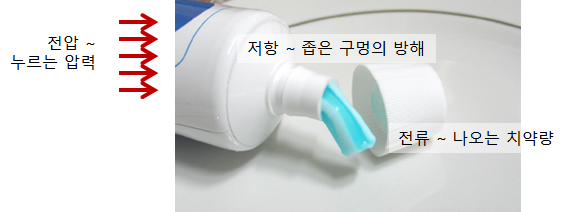# Ohm’s Law

Ohm’s Law

When you connect the bulb and the battery with a wire, the bulb lights up. This is because free electrons with negative charge (-) move along the wire. At this time, it is considered that the (+) charge moves in the opposite direction and is called the electric current.
It is easy to understand if the current is likened to the flow of water. The current can be compared to the amount of water flowing per second. In order for water to flow, pressure must be applied somewhere, which can be compared to voltage. The property that limits the flow of water is resistance.The intensity of the current flowing through the circuit is proportional to the voltage and inversely proportional to the resistance. Ohm’s law is the relationship between voltage V, resistance R, and current I.

\begin{align} Current\; &∝\; \frac { Voltage }{ Resistance } \\ I\; &∝\; \frac { V }{ R } \end{align}

The analogy of Ohm’s law

Consider the case of squeezing toothpaste. The force that presses the toothpaste is the voltage, the narrow hole of the toothpaste is the resistance, and the amount of toothpaste is thought of as the current.If you press the toothpaste tube strongly, toothpaste will come out well. ($$Current ∝ Voltage$$)
The narrower the hole, the less the toothpaste will come out. ($$Current\; \propto \; \frac { 1 }{ Resistance }$$)

Electric shock

Electric shock is caused by current flowing in the body. If there is a voltage difference between one part of the body and the other part, an electric shock will occur.
The current has the property of passing through a place where it is easy to flow (where the electric resistance is small). Normally, there is a large resistance between the ground and the legs, so there is not a large current flowing to injure the body. However, if you are wet, your body’s electrical resistance will be small, allowing a large current to flow. Never touch the electric appliance while bathing..

• 0.001 A ~ You can feel the flow of current in your body.
• 0.005 A ~ It is painful.
• 0.01 A ~ It causes muscle contraction.
• 0.015 A ~ Muscles will be paralyzed.
• 0.07 A ~ It affects the heart. Larger currents are fatal. (Conceptual physics 7th Edition – Paul G. Hewitt)